## Welcome to the World of Modelling and Simulation

### What is Modelling?

This blog is all about system dynamics modelling and simulation applied in the engineering field, especially mechanical, electrical, and ...### System Identification and Model Reference Adaptive Control

Part 1: System Identification

Principle of Least Squares
Gauss formulated the principle of least squares method and according to his postulates “The sum of the squares of the differences between the actually observed and the computed values, multiplied by numbers that measure the degree of precision, is a minimum” 
Generally, least square method may be applied to a variety of systems; however it is very efficient in the following mathematical model :

Where, y is the observed variable and 𝞡s are the parameters of the above mathematical model to be determined, and 𝞍s are the known function of the model which may depend on other variables.
The known model functions and determinable system parameters may be written in the following matrix forms:

The model is indexed by the variable i which often represents the time.
The least square lost function V(𝚹, t)  may be expressed by the following expression :

The lost function in equation (4) is minimal for the estimate if,

If the matrix is nonsingular, then the minimal is unique which is given by following equation:

Now, a MATLAB algorithm can be written based on the above equation. In this lab, a MATLAB program is given in the course website  which is slightly modified to account for the DC motor first order system.
The DC motor mathematical model can be given by the following equation:

Where, τ is the time constant and C is the DC gain. This is the second order differential equation. For the simple open loop system, this equation can be assumed by a first order equation by following consideration.

Where, ɷ is the angular speed of the DC motor. So, the above equation is simplified as:

This difference equation is inserted in the mathematical model equation. Here, the unknown parameters are C and τ which will be estimated by the following least square algorithm. To initialize the algorithm, first these parameters are assumed.

MATLAB Program for DC Motor First Order Parameters Estimation

close all
clear all
clc
clear
kmax = input('number of data points: ') ;
dd = input('enter delay: ') ;
u = randn(200,1) ;
y = zeros(200,1) ;
P = 10000*eye(2) ;
np = norm(P)*ones(kmax,1) ;
thetar = zeros(2,kmax) ;
for k=dd+3:200,
y(k) = -0.1*y(k-1)+0.5*u(k-1) ;
end
for k=dd+3:kmax,
phit(k,:) = [ y(k-1) u(k-dd-1) ] ;
Y(k-1,:) = y(k) ;
Phi(k-1,:) = phit(k,:) ;

np(k) = norm(P) ;
P = inv(inv(P) + phit(k,:)'*phit(k,:)) ;
thetar(:,k) = thetar(:,k-1) + P*phit(k,:)'*(y(k)-phit(k,:)*thetar(:,k-1)) ;

end
theta = inv(Phi'*Phi)*Phi'*Y

Program Outputs:

>> modifiedid
number of data points: 50
enter delay: 1

theta =

-1.4574
0.6470

A very rough analysis on the parameters is taken in this study. This analysis gives the understanding of the parameters estimation in practical fields.

Part 2: Model Reference Adaptive Control (MRAC)
In this section, the effect of the simulation time on the model reference adaptive control system is observed. The model reference adaptive control model is given in the course website  from which the simulations are performed. In this case, the system has an ordinary feedback loop composed of the process and the controller and another feedback loop which changes the controller parameters. The parameters are changed on the basis of feedback from the error, which is the difference between the output of the physical system and the reference model.
By the following simulation results, the effect of the length of the simulation time is observed on the model. The colors for the three signals in simulation refer: input to the system (yellow), physical plant response (purple) and reference model response (light blue). With the increase in simulation time sequentially, it is realized by observing the following figures that the drifts in the plant response and controller signal increase. The possible reason may be the presence of integrators in the controller which increases control gains over the time.Figure 1: Showing the model output (light blue), plant output (purple) and the input (yellow) when simulation time is 60s.Figure 2: Showing the controller signal when simulation time is 60s.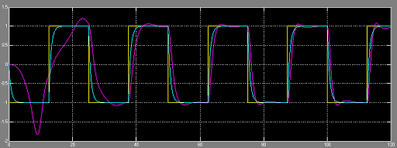Figure 3: Showing the model output (light blue), plant output (purple) and the input (yellow) when simulation time is 120s.Figure 4: Showing the controller signal when simulation time is 120s.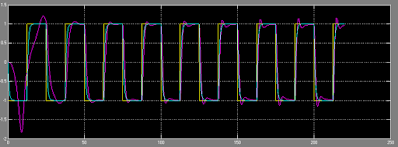Figure 5: Showing the model output (light blue), plant output (purple) and the input (yellow) when simulation time is 220s.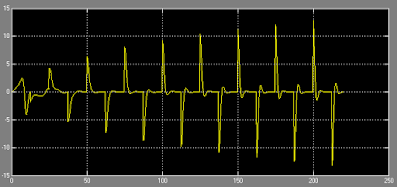Figure 6: Showing the controller signal when simulation time is 220s.Figure 7: Showing the model output (light blue), plant output (purple) and the input (yellow) when simulation time is 420s.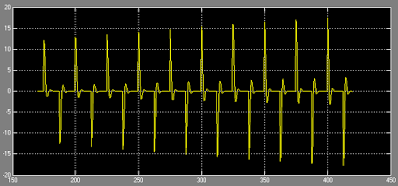Figure 8: Showing the controller signal when simulation time is 420s.Figure 9: Showing the model output (light blue), plant output (purple) and the input (yellow) when simulation time is 820s.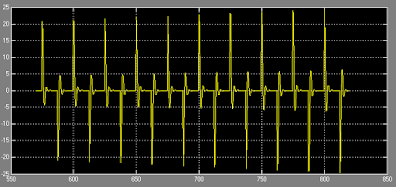Figure 10: Showing the controller signal when simulation time is 820s.

References
 Adaptive control / Karl Johan Åström, Björn Wittenmark.—2nd ed., Addison-Wesley Publishing Company, 1995.
 Dr. Jeff Pieper, System Identification MATLAB Program, March 2014.
 Dr. Jeff Pieper, SIMULINK Model for MRAC, March 2014